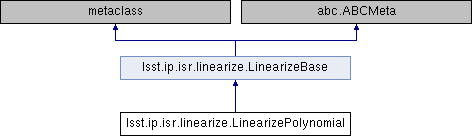lsst.ip.isr.linearize.LinearizePolynomial Class Reference
Inheritance diagram for lsst.ip.isr.linearize.LinearizePolynomial:## Public Member Functions

def __call__ (self, image, **kwargs)

## Static Public Attributes

string LinearityType = "Polynomial"

## Detailed Description

Correct non-linearity with a polynomial mode.

corrImage = uncorrImage + sum_i c_i uncorrImage^(2 + i)

where c_i are the linearity coefficients for each amplifier.
Lower order coefficients are not included as they duplicate other
calibration parameters:
"k0"
A coefficient multiplied by uncorrImage**0 is equivalent to
bias level.  Irrelevant for correcting non-linearity.
"k1"
A coefficient multiplied by uncorrImage**1 is proportional
to the gain.  Not necessary for correcting non-linearity.


Definition at line 595 of file linearize.py.

## ◆ __call__()

 def lsst.ip.isr.linearize.LinearizePolynomial.__call__ ( self, image, ** kwargs )
Correct non-linearity.

Parameters
----------
image : lsst.afw.image.Image
Image to be corrected
kwargs : dict
Dictionary of parameter keywords:
"coeffs"
Coefficient vector (list or numpy.array).
If the order of the polynomial is n, this list
should have a length of n-1 ("k0" and "k1" are
not needed for the correction).
"log"
Logger to handle messages (logging.Logger).

Returns
-------
output : tuple [bool, int]
If true, a correction was applied successfully.  The
integer indicates the number of pixels that were
uncorrectable by being out of range.


Reimplemented from lsst.ip.isr.linearize.LinearizeBase.

Definition at line 612 of file linearize.py.

612  def __call__(self, image, **kwargs):
613  """Correct non-linearity.
614
615  Parameters
616  ----------
617  image : lsst.afw.image.Image
618  Image to be corrected
619  kwargs : dict
620  Dictionary of parameter keywords:
621  "coeffs"
622  Coefficient vector (list or numpy.array).
623  If the order of the polynomial is n, this list
624  should have a length of n-1 ("k0" and "k1" are
625  not needed for the correction).
626  "log"
627  Logger to handle messages (logging.Logger).
628
629  Returns
630  -------
631  output : tuple [bool, int]
632  If true, a correction was applied successfully. The
633  integer indicates the number of pixels that were
634  uncorrectable by being out of range.
635  """
636  if not np.any(np.isfinite(kwargs['coeffs'])):
637  return False, 0
638  if not np.any(kwargs['coeffs']):
639  return False, 0
640
641  ampArray = image.getArray()
642  correction = np.zeros_like(ampArray)
643  for order, coeff in enumerate(kwargs['coeffs'], start=2):
644  correction += coeff * np.power(ampArray, order)
645  ampArray += correction
646
647  return True, 0
648
649

## ◆ LinearityType

 string lsst.ip.isr.linearize.LinearizePolynomial.LinearityType = "Polynomial"
static

Definition at line 610 of file linearize.py.

The documentation for this class was generated from the following file:
• /j/snowflake/release/lsstsw/stack/lsst-scipipe-0.7.0/Linux64/ip_isr/22.0.1-27-g97737f7+2a20fdde0d/python/lsst/ip/isr/linearize.py